# The accounting equation

• Study Reminders

We'll email you at these times to remind you to study

You can set up to 7 reminders per week

#### You're all set

We'll email you at these times to remind you to study

Monday

Tuesday

Wednesday

Thursday

Friday

Saturday

Sunday

• Study Notes
• Discuss This Topic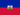Petuel E. 1 0 It's strongly important to make sure that the accounting equation is a state of balance . And of course the left hand side of the seesaw is the debit side and the right hand side is the credit side. so There must be equal weight each end.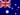Wendy C. 1 0 Initially assets are equal to liabilities plus owner's equity (proprietorship). Once the business commences operations this equation extends to Assets + Expenses = Revenue + Liabilities + Owner's equity (proprietorship). A + E = R + L + P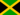Anton A. 0 0 What is the accounting equation about?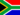Samuel F. 1 0 Is it possible for owner's equity to be a negative amount?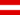Harrison A. 0 0 What is net income ?Zachary B. 0 0 What is the accounting equation?Zachary B. 0 0 What is the accointing equation?Diamond T. 1 0 The negative amount of owner's equity also means that the company's balance sheet will report liability amounts greater than the amount of assets. The company could operate under those conditions if its assets are turning to cash before the liabilities need to be paidDouglas R. 1 0 Equality on both credit and debit makes a smile face.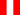Erick V. 0 0 compensación y equilibrio contable
• Text Version